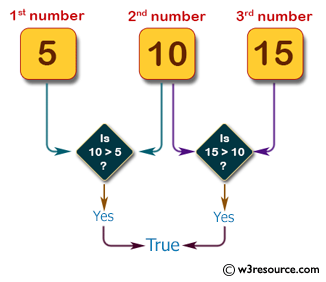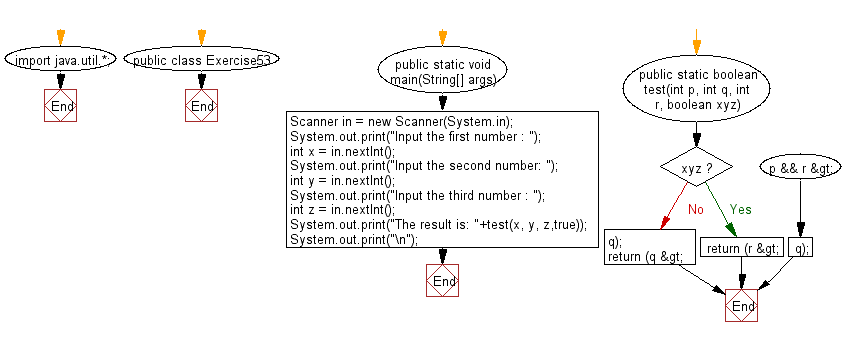﻿ Java: If 2 or more of 3 integers have same rightmost digit# Java Exercises: Accepts three integers from the user and return true if the second number is greater than first number and third number is greater than second number

## Java Basic: Exercise-53 with Solution

Write a Java program that accepts three integers from the user and return true if the second number is greater than first number and third number is greater than second number. If another variable "abc" is true second number does not need to be greater than first number.

Sample Solution:

Java Code:

``````import java.util.*;
public class Exercise53 {
public static void main(String[] args)
{
Scanner in = new Scanner(System.in);
System.out.print("Input the first number : ");
int x = in.nextInt();
System.out.print("Input the second number: ");
int y = in.nextInt();
System.out.print("Input the third number : ");
int z = in.nextInt();
System.out.print("The result is: "+test(x, y, z,true));
System.out.print("\n");
}

public static boolean test(int p, int q, int r, boolean xyz)
{
if(xyz)
return (r > q);
return (q > p && r > q);
}
}
```
```

Sample Output:

```Input the first number : 5
Input the second number: 10
Input the third number : 15
The result is: true
```

Pictorial Presentation:Flowchart:Java Code Editor:

What is the difficulty level of this exercise?

Test your Programming skills with w3resource's quiz.

﻿

## Java: Tips of the Day

countOccurrences

Counts the occurrences of a value in an array.

Use Arrays.stream().filter().count() to count total number of values that equals the specified value.

```public static long countOccurrences(int[] numbers, int value) {
return Arrays.stream(numbers)
.filter(number -> number == value)
.count();
}
```

Ref: https://bit.ly/3kCAgLb